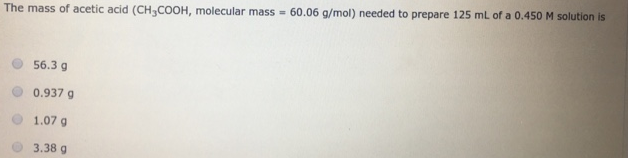Chemistry Molarity Solution: The mass of acetic acid (CH3COOH, molecular mass =...

# Solution: The mass of acetic acid (CH3COOH, molecular mass = 60.06 g/mol) needed to prepare 125 mL of a 0.450 M solution isa. 56.3 gb. 0.937 gc. 1.07 gd. 3.38 g

Problem

The mass of acetic acid (CH3COOH, molecular mass = 60.06 g/mol) needed to prepare 125 mL of a 0.450 M solution is

a. 56.3 g

b. 0.937 g

c. 1.07 g

d. 3.38 gView Complete Written Solution